+
Solving Linear Equations and Inequalities
0
of 0 possible points

Author: Sophia Tutorial
##### Description:

Multiply binomial radical expressions using FOIL.

(more)

Sophia’s self-paced online courses are a great way to save time and money as you earn credits eligible for transfer to many different colleges and universities.*

No credit card required

46 Sophia partners guarantee credit transfer.

299 Institutions have accepted or given pre-approval for credit transfer.

* The American Council on Education's College Credit Recommendation Service (ACE Credit®) has evaluated and recommended college credit for 33 of Sophia’s online courses. Many different colleges and universities consider ACE CREDIT recommendations in determining the applicability to their course and degree programs.

Tutorial
what's covered
1. Review of Distributive Rule and FOIL with Integers
2. Multiplying Radical Expressions using Distribution and FOIL

# 1. Review of Distributive Rule and FOIL with Integers

The distributive rule allows us to distribute an outside factor into all terms of another factor. For example: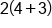Distribute 2 into 4 and 3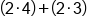Multiply inside the parentheses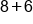Add 8 to 6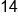Our Solution

If we have to factors in the form (a+b), we can use the distributive property in a different way, commonly referred to as the FOIL method.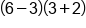Apply steps to FOIL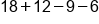Add and subtract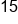Our Solution

# 2. Multiplying Radical Expressions using Distribution and FOIL

The distributive rule and FOIL method can be applied to multiply expressions with radicals as well. First, we will look at an example of distribution, where two identical radicals are multiplied together.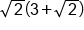Distribute the square root of 2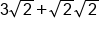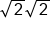simplifies to the integer 2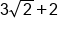Our Solution

We can also use the FOIL method to distribute across two binomials in multiplication when there are radicals. This is illustrated in the following example: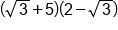Apply the steps to FOIL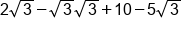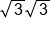simplifies to the integer 3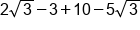Combine like terms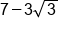Our Solution

big idea
The distributive rule and the FOIL method can be applied to expressions containing radicals as well. When two identical square roots are multiplied by each other, it evaluates to the expression underneath the square root. This property also applies to other roots, such as cube roots, but the identical radical needs to be multiplied by itself 3 times, and so on.

term to know
FOIL
An acronym to remember the steps for distributing factors in binomial multiplication: first, outside, inside, last.

summary
A review of the distributive property and FOIL allows us to distribute outside factors into all terms of another factor. A helpful hint when multiplying radical expressions using distribution and FOIL is to remember that multiplying 2 square root terms together will cancel out the square root operation. For example, the square root of 8 times the square root of 8 is just 8.

Terms to Know
FOIL

An acronym to remember the steps for distributing factors in binomial multiplication: first, outside, inside, last.

Formulas to Know
FOIL MethodRating